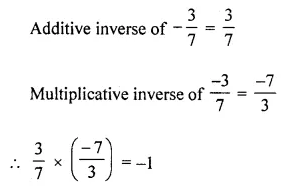# ML Aggarwal Class 8 Solutions for ICSE Maths Chapter 1 Rational Numbers Ex 1.3

## ML Aggarwal Class 8 Solutions for ICSE Maths Chapter 1 Rational Numbers Ex 1.3

Question 1.
Multiply and express the result in the lowest form: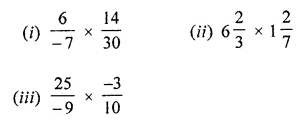Solution: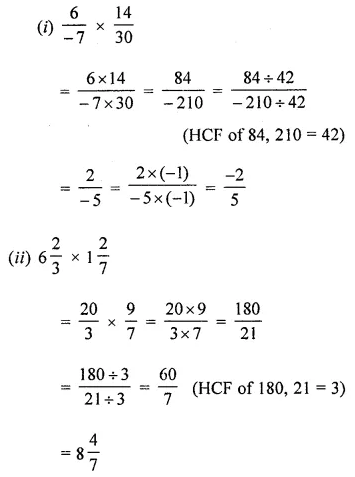Question 2.
Verify commutative property of multiplication for the following pairs of rational numbers:
(i) $$\frac { 4 }{ 5 }$$ and $$\frac { -7 }{ 8 }$$
(ii) 13$$\frac { 1 }{ 3 }$$ and 1$$\frac { 1 }{ 8 }$$
(iii) $$\frac { -7 }{ -20 }$$ and $$\frac { 5 }{ -14 }$$
Solution: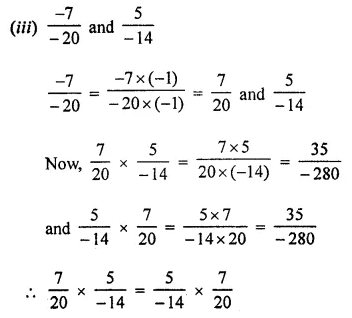Question 3.
Verify the following and name the property also:Solution: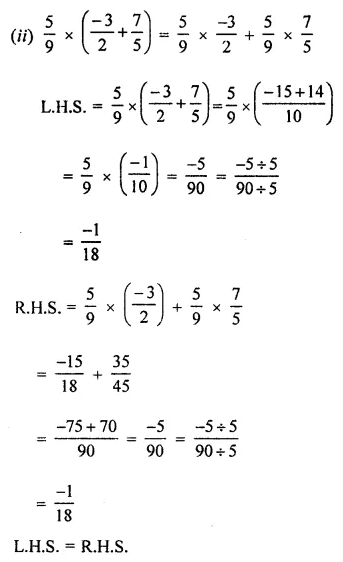Question 4.
Find the multiplication inverse of the following: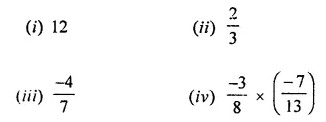Solution:Question 5.
Using the appropriate properties of operations of rational numbers, evaluate the following: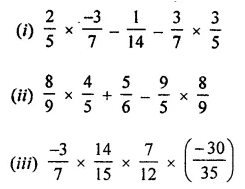Solution: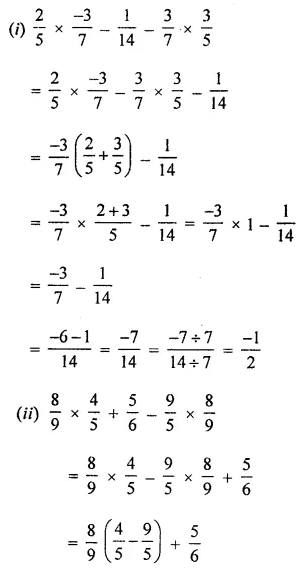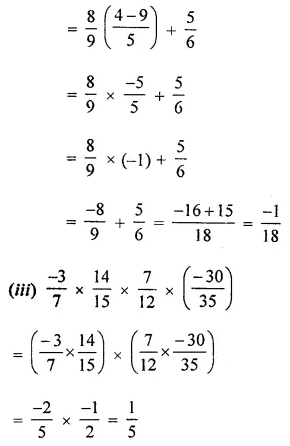Question 6.
If p = $$\frac { -8 }{ 27 }$$, q = $$\frac { 3 }{ 4 }$$ and r = $$\frac { -12 }{ 15 }$$, then verify that
(i) p × (q × r) = (p × q) × r
(ii) p × (q – r) = p × q – p × r
Solution: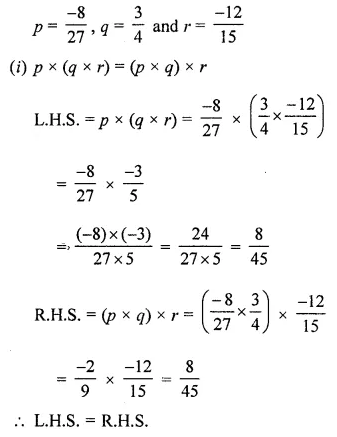Question 7.
Fill in the following blanks:(ix) The reciprocal of 0 is …….
(x) The numbers ……… and ……. are their own reciprocals.
(xi) If y be the reciprocal of x, then the reciprocal of y2 in terms of x will be ………
(xii) The product of a non-zero rational number and its reciprocal is ………
(xiii) The reciprocal of a negative rational number is ………..
Solution: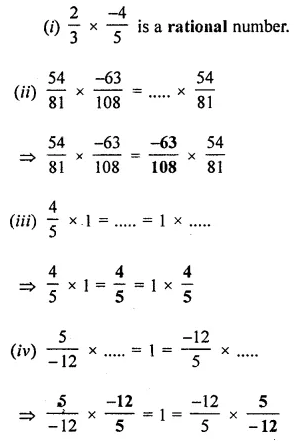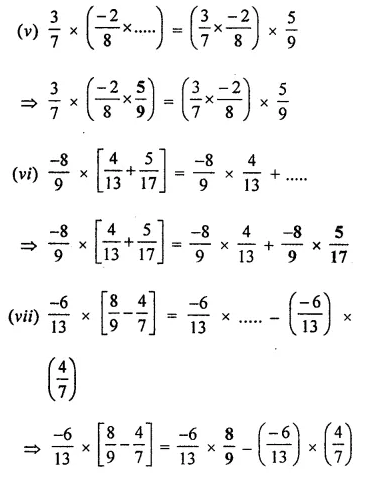Question 8.
If $$\frac { 4 }{ 5 }$$ the multiplicative inverse of -1$$\frac { 1 }{ 4 }$$ ? Why or why not?
Solution: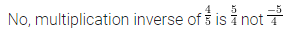Question 9.
Using distributivity, findSolution: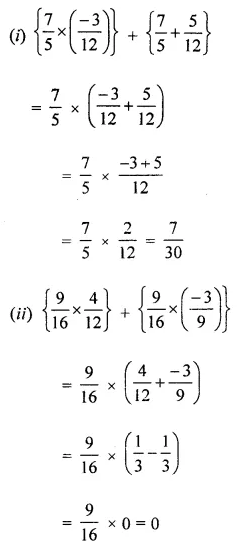Question 10.
Find the sum of additive inverse and multiplicative inverse of 9.
Solution: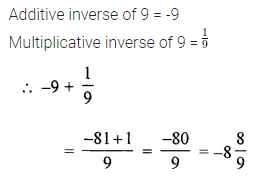Question 11.
Find the product of additive inverse and multiplicative inverse of $$\frac { -3 }{ 7 }$$
Solution: